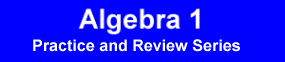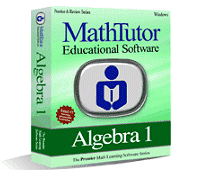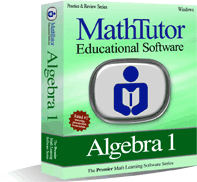Award-Winning

# Algebra 1 Academic Instructional Review and Practice SoftwareDESCRIPTION
ALGEBRA 1 is an acclaimed software package designed to help students master problem solving through practice and review.
Designed by math educators, ALGEBRA 1 provides carefully designed practice and drill exercises tailored to each student's ability.
The program uses repetitive practice to reinforce the concepts students are learning in elementary algebra. Problems are randomized and based on students' skill levels.
ALGEBRA 1 provides interactive, step by step problem explanations enabling students to learn how to apply the concepts they have learned to problem solving. Feedback and recordkeeping help students gain confidence and see the progress they have made.
Students master problem solving through exercises that progress gradually from the simpler to the more challenging.
• Elementary algebra drill and practice software developed by math curriculum specialists
• Dynamic, interactive algebra 1 problem solving exercises tailored to student skill levels
• Proven results boosting student proficiency in problem solving and comprehensionTOPICS COVERED
Review of Arithmetic
• Basic Rules of Arithmetic
• Add., Subtr., Mult., Division of Fractions
• Comparison of Fractions
• Interpretation of Fractions
• Arithmetic Comparisons
• Scientific Notation
Simple Algebraic Operations
• Addition and Subtr. of Polynomials
• Multiplication of Polynomials
• Elementary Factoring I
• Elementary Factoring II
• Addition and Subtr. of Rational Expressions
• Solving Simple Algebraic Equations I
• Solving Simple Algebraic Equations II
• Units of Measure
• D = RT
• Elementary Word Probs I: Linear Eqns
• Elementary Word Probs II: Quad. Eqns
Operations

• Intermediate Factoring
• Finding the Roots of a Quadratic Equation
• Combining Algebraic Expressions I
• Combining Algebraic Expressions II
• Simplifying Radicals I
• Simplifying Radicals II
• Exponent Rules
• Pythagorean Theorem
• Intermediate Word Problems I
• Intermediate Word Problems II
Functions and Miscellaneous Topics
• Reading and Interpreting Tables
• Simple Word Problems
• Coordinate Geometry
• Lines in the Plane
• Equation of a Straight Line
• Solving an Inequality
• Absolute Value Function
• Determining a Function
• Direct and Inverse Proportions
• Word Problems: Absolute Value